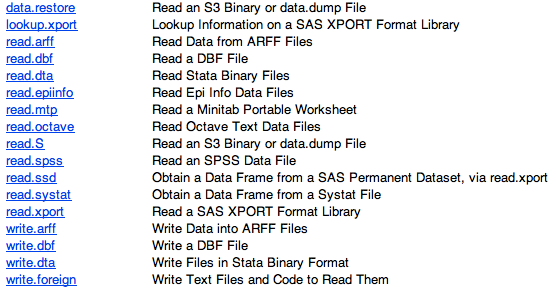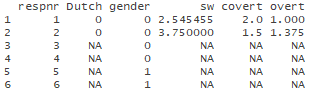# R: how to get started

## Solutions

Developed by Naomi Schalken, Lion Behrens and Rens van de Schoot

This tutorial expects:

• Basic knowledge of correlation and regression

In this tutorial, the reader will be guided through the process of downloading R and its working environment RStudio as well as first statistical computations that can serve as a reference for further exploring the R language. Throughout this tutorial we will use a dataset from Van de Schoot, van der Velden, Boom & Brugman (2010). Using multiple regression, we will predict adolescents’ socially desirable answering patterns (sw) from overt (overt) and covert (covert) antisocial behaviour. For more information on the sample, instruments, methodology and research context we refer the interested reader to the paper (see references). Here we will focus on data-analysis only.

### Preparation - Installing R and RStudio

1. To install R:
1. Go to http://www.r-project.org/ -> CRAN -> select your country
4. R will now be installed
2. To install RStudio:
2. Go to the bottom of the page to -> Installers for Supported Platforms -> and select the download for your operating system
3. RStudio will now be installed

Working with R is now possible via RStudio, so opening RStudio is enough.

### Exercise 1 - explore data in R

Some general remarks: we will frequently ask you to “run a command” in R. You can do so by pressing Ctrl+Enter after you’ve typed/pasted a section of R code. You may assume the command was processed accordingly when no errors are reported and a new prompt appears.

Exercise 1a. Importing .sav data in R

Usually, you are starting with a SPSS dataset stored as a .sav file. To get to your .sav file, download popular_regr_1.xlsx, open it with SPSS and save it as you would usually do it under the name `popular_regr_1.sav`. Then, after opening R, we start by importing our .sav file in three steps:

1. Set the working directory so that R knows where to look for the .sav file. To do this, type the setwd(“”) command where you enter your working directory between quotation marks. Hint: right-clicking the SPSS file `popular_regr_1.sav` on your computer and asking for Properties will give you the working directory. Run the setwd() command by pressing enter. Attention: To enable R to find your file, all backslashes need to be changed into normal slashes (“/”). Your setwd(“”) command could for example look like this:
``````setwd("C:/Users/ExampleUser/Desktop")
``````

Now R knows where to find your .sav file.

1. Activate the in-built foreign package by running
``library(foreign)``

This opens up options that we need in step 3. The foreign package assists R in importing datafiles from SPSS, STATA, SAS, MiniTab et cetera:Question: Which command would you use to import the SPSS-file?

1. Import the .sav file with the following command:
``popular <- read.spss("popular_regr_1.sav", to.data.frame = TRUE)``

You can ignore the following warning:
Warning message: In read.spss(“popular_regr_1.sav”, to.data.frame = TRUE) : popular_regr_1.sav: Unrecognized record type 7, subtype 18 encountered in system file

To see if the data was imported correctly we can use the following function:

``head(popular)``

This will show the data-file for the first 6 subjects. If you run this command, you should see the following:Note the coding of Dutch is 0=yes, 1=no and gender is coded 0=boy, 1=girl. As you can see, some of the data is missing (NA; Not Available). If this is not the case (or in general if you need to code missing values) you can manually identify missing values by running the following R command, where -999 (or 99) is the value used in SPSS to denote missing data:

``popular[popular==-999] <- NA``

Finally, we need to attach the data to use the variable names of sw, overt and covert directly by using

``attach(popular)``

Note that the attach command is merely a technical step; no output is expected.

Exercise 1b. Looking at descriptive results using R

Let’s explore the R environment in closer detail. A general function that’s useful for obtaining descriptives is

``summary(popular)``

Run this command to obtain means and other useful info for every variable separately. Now let’s look at the data graphically, for example by means of a boxplot:

``boxplot(sw)``

Finally, let’s consider bivariate relations by looking at the correlations like we did in SPSS using:

``cor(popular[,(4:6)], use = "pairwise")``

In this command we call the cor (=correlation) function and say that we want to use columns 4 through 6 of the data, and all rows. To deal with the missing data, for now, we ask for pairwise correlations.

Question: Compare your results to the results obtained in SPSS: How to get stared. Are the results similar? If not, can you explain the differences between the R output and the SPSS output?

Note that the correlation between Covert and Overt is estimated to be -0.3335563 in R and -0.334 in SPSS. This can be explained by differences in rounding; SPSS obtains -0.334 by rounding the third decimal whereas R displays more decimals of the correlation, leaving the third decimal at ‘3’. If there are any other differences between the correlations you obtained with SPSS and R it is likely that something went wrong.

### Exercise 2 - Multiple Regression in R

Just as in any other statistical software package, you can use R to run (and program) multivariate statistical analyses. Consider the linear regression example conducted in SPSS: how to get started. The task of this exercise is to reproduce the results achieved in SPSS using R.

In order to do that, start by defining the regression model.

``model  <- 'sw ~ overt + covert'   ``

Here, we create something named “model”" that we call later on. This “model” is denoted by a bunch of characters (called a string) between single quotation marks. This string is assigned by the arrow symbol (<-). The string itself says that sd, the dependent variable, is regressed on (~) the linear combination of overt and covert. The intercept is not included in the equation as R automatically accounts for the existence of an intercept in linear regression. To run the model and store the output in an R object we can run the following code:

``fit <- lm(model, data = popular)  ``

``summary(fit)   ``

Question: How would you interpret the results? How do they compare to the results that can be found in SPSS: How to get started?

### References

Van de Schoot, R., van der Velden, F., Boom, J. & Brugman, D. (2010). Can at Risk Young Adolescents be Popular and Antisocial? Sociometric Status Groups, AntiSocial Behavior, Gender and Ethnic Background. Journal of Adolescence, 33, 583-592.

### Now that you got started with R, why don't you also get started with:

MPLUS

How to get started

Blavaan

How to get started

Lavaan

How to get started

RJAGS

How to get started

JASP

How to get started